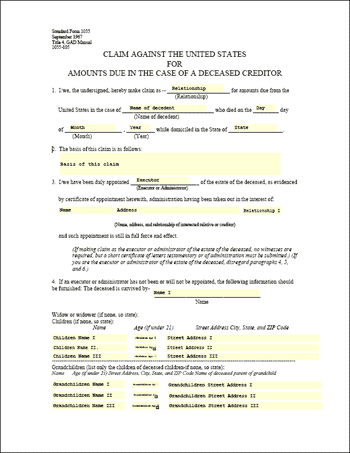eForm Fields ExampleThis example shows how to change the values of eForm fields. In this example we simply replace each of the fields in a form with the name of that field.Src

 First we create an ABCpdf Doc object and read in our template form. [C#] ```Doc theDoc = new Doc(); theDoc.Read(Server.MapPath("../mypics/form.pdf"));``` [Visual Basic] ```Dim theDoc As Doc = New Doc() theDoc.Read(Server.MapPath("../mypics/form.pdf"))```We iterate through each of the top level fields. For each field we set the value of the field to be equal to the value of the name. [C#] ```string[] theNames = theDoc.Form.GetFieldNames(); foreach (string theName in theNames) {   Field theField = theDoc.Form[theName];   theField.Value = theField.Name; }``` [Visual Basic] ```Dim theNames As String() = theDoc.Form.GetFieldNames() Dim theName As String For Each theName In theNames   Dim theField As Field = theDoc.Form(theName)   theField.Value = theField.Name Next```Finally we save. [C#] `theDoc.Save(Server.MapPath("eformfields.pdf"));` [Visual Basic] `theDoc.Save(Server.MapPath("eformfields.pdf"))`Given the following document.form.pdf This is the kind of output you might expect.eformfields.pdf